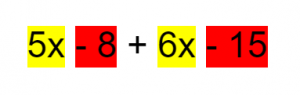# Simplifying Algebraic Expressions (Algebra 2)

 Would You Rather Listen to the Lesson?In Algebra 2, algebraic expressions are one of the most important concepts students encounter. They learn how to simplify them or rewrite them in a simplified way. To achieve this, they apply the properties of real numbers and produce an equivalent form of an expression.

Indeed, all the x’s and y’s can come across as tricky, but they’re certainly not impossible to learn! Math teachers can adopt various strategies to support students in becoming fluent in simplifying algebraic expressions. Read on to find out more.

## Algebraic Expressions Definition

For starters, remind students of the definition of an algebraic expression. By now, most students should be able to tell you that an algebraic expression is a mathematical expression comprising constants, variables, and operations (such as addition, subtraction, multiplication, etc.).

Give an example, such as 8x + 4. This is a simple algebraic expression, as it contains two constants (the number 8 and 4 which don’t change), a variable (the letter x, which can change), and an operation (subtraction).Remind children that when algebra problems come as word problems, we must transform these words into algebraic expressions, known as writing algebraic expressions. Substituting a number for each variable and doing the operation is called evaluating algebraic expressions.

Finally, explain that simplifying an algebraic expression means that we’re turning a long expression into something that we can easily make sense of. Then, you can provide an example. For instance, you can say that by using simplification, we can turn 2x 5y – y2 into 4xy.

You may also want to check out our article on writing and evaluating algebraic expressions.

## Teaching How to Simplify Algebraic Expressions

### Bell-Work Activity

In order for students to be able to simplify an algebraic expression, they need to have a solid grasp of the properties of real numbers. This is why it’s advisable to do a few exercises so that you can review previously acquired knowledge in this regard.

You can use this simple Bell-Work Worksheet for this purpose. Print out a sufficient number of copies and distribute them to each student. The worksheet contains exercises on properties of real numbers, such as the distributive property, the commutative property, associative property, etc.

## Simplifying Algebraic Expressions

Start by writing an easier example on the whiteboard, such as:

5x – 8 + 3 (2x – 5) = ?

Explain that by applying the distributive property, we’ll get the following expression:

5x – 8 + 6x – 15

Point out to students that when we simplify an algebraic expression, we also need to combine similar terms. Illustrate the like expressions in the above example. You can use a marker to highlight the like expressions in different colors. That is:That means that:

5x + 6x = 11x

– 8 – 15 = – 23

Or:

11x – 23

You can use this video in your classroom to present the process of simplifying algebraic expressions. It starts with simpler examples and gradually moves on to more complicated expressions.

Finally, distribute this Guided Notes Worksheet for even more challenging expressions.

## Activities to Practice Simplifying Algebraic Expressions

In Algebra 2, algebraic expressions and their simplification can be mastered with the help of different engaging activities in your classroom. Use the following activities for group work, or adjust them to individual work if you’re a homeschooling parent.### Online Game

Divide children into pairs and explain that they’ll play an online game to practice how to simplify algebraic expressions. Make sure that each student has a suitable device.

Explain to students that each person in the pair will play the game individually on their device. The game consists of answering different math questions related to simplifying algebraic expressions, such as:

Simplify the following expression:

-3(x + y) + 5(x – y)

The game usually offers multiple answers from which the student needs to select one. For each question that a student answers correctly, they score points. In the end, the students compare their scores and the one with the highest score wins the game.

### Group Work

To implement this activity in your classroom, print out enough copies of this Assignment Worksheet, depending on the number of students in your class. Then, divide students into groups of three or four and hand out the copies.

The worksheet contains math problems connected to simplifying algebraic expressions or writing an algebraic expression to the given verbal expression. Each group works together to solve the math problems in the worksheet.

In the end, each group selects one representative to present their answers in front of the class, as well as explain how they reached a specific solution. This activity will encourage cooperation within a group, as well as reinforce presentation skills.

## Before You Leave…

In algebra 2, algebraic expressions and the process of simplifying them can be an engaging experience for children if you’re equipped with the right resources!

So, if you liked our math strategies in this article, make sure to check out our resources on simplifying algebraic expressions:

To get the Editable versions of these files, join us inside the Math Teacher Coach Community: